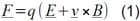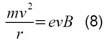Students are famous for many things. Drinking too much, hoarding traffic cones and sleeping through lectures are all stereotypical student sports. However, it is hard to imagine most students making it to graduation day without their trusty microwave. From ready meals and speedy jacket potatoes, to warming up last night’s pizza and cooking chicken soup to combat fresher’s flu, microwaves are a staple of student life. Yet whether its user is studying modern history or media studies, it is mathematics that’s crucial to the design of the microwave.

### The Magnetron

A magnetron within the microwave oven generates electromagnetic radiation at microwave frequencies, hence its name. The microwaves are created when electrons interact with the magnetic field created by the magnets within it.

The specific wavelength of microwaves created within the oven is 12.2cm. The reason for this is that at this wavelength the radiation excites water molecules within the food. Even seemingly dry food has a relatively high water content as fats and sugars contain water too. The water molecules vibrate and heat up the food. The reason microwaves cook food much more quickly than conventional ovens is that they cook the food from “the inside out”. What’s more, 12.2cm microwave radiation has little effect on materials like glass and plastic. This allows the food to be contained.

However, to produce microwaves of 12.2cm, the strength of the magnet needs to be precise. It is calculated by starting with the Lorentz Force Law:where F is the force experienced by a particle of charge q, travelling at a velocity v, in a combined electric and magnetic field, the strengths of which are E and B respectively.

For an electron in a magnetic field with no electric field:

E = 0 and q = e (the charge on the electron)

This gives:This means that F is perpendicular to v, which is the same as the velocity of the Earth being perpendicular to the force of gravity towards the Sun: the result is circular motion. Therefore, an electron in a purely magnetic field moves in a circle.

Writing the cross product out in full gives:v and B are perpendicular too, and so the cross product just reduces to vB. This gives the force as:But Newton’s second law of motion also says that:where m is the mass and a is acceleration.

The acceleration of body moving in a circle is:where v is the velocity of the orbiting particle and r is the radius of the orbit.

This gives the force towards to centre of the circle asEquating this to equation (4) gives:Re-arranging in terms of r gives:The angular frequency, ?, of the electron can then be given by two different equations:where f is linear frequency.

Substituting in for r from equation (9) gives:Microwaves travel at the speed of light and so we can use the fact that:where c is the speed of light in a vacuum and ? is the wavelength.

Substituting into equation (11) provides us with:Re-arranging for B gives:Everything on the right hand side is a fundamental constant except for the wavelength, λ. This means the magnetic field strength is inversely proportional to the wavelength. Plugging in a value of 0.122 metres for λ and the mass of the electron for m, the value of B is 0.088 Tesla.

Finally, even if you have the right magnetic field strength, a microwave is useless unless the food is rotated inside. This is because of the way the oven exploits the microwaves the magnetron creates. The walls of the microwave are made of metal which reflect the waves back towards the centre. These waves interfere with one another inside the microwave – sometimes destructively and sometimes constructively. When the inference is constructive you get “hot spots”. These hot spots can only heat the food evenly if it is rotated through these stationary locations.

So next time you devour a curry ready meal, spare a thought for the mathematics behind your microwaved Madras.